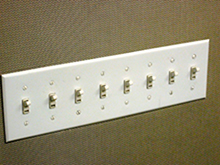# The Hardest Prisoner Problem Ever (Part 3)

Logic Level 4Do not attempt this problem until you have solved
The Hardest Prisoner Problem Ever (Part 1) and
The Hardest Prisoner Problem Ever (Part 2).

This problem is the same as Part 1 except:

• you have 8 switches in the room instead of 1
• you have on the order of 1,000,000,000,000,000,000,000,000 ($10^{24}$ prisoners aka a yotta-prisoner) prisoners instead of 6.
• your goal now is to find an algorithm that will win as quickly as possible.

Assuming that the prisoners use the fastest possible strategy, approximately how long will the game take as a function of the number of prisoners, $n$? (i.e. what is O(fastest strategy)?)

×